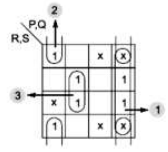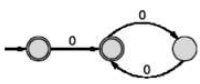# GATE Solved Paper 2017-19 - GATE 2018

Here, m denotes a minterm and d denotes a don’t care term. The number of essential prime implicants of the function F is ______ .

Note: Numerical Type question.

• A

1• B

2• C

3• D

4• Option : C
• Explanation :
F(P, Q, R, S) = Σm(0, 2, 5, 7, 9, 11) + d(3, 8, 10, 12, 14)Number of EPI = 3

• A

219• B

104• C

115• D

220• Option : A
• Explanation :
Given, total number of instructions (n) = 100
Number of stages (k) = 5
Since, if n instructions take c cycle, so (c-1) stalls will occur for these instructions.
Therefore, the number of clock cycles required = Total number of cycles required in general case + Extra cycles required (here, in PO stage)
= (n + k – 1) + Extra cycles
= (100 + 5 -1) + 40*(3-1)+35*(2-1)+20*(1-1)
= (100 + 4) + 40*2+35*1+20*0
= 104 + 115
= 219 cycles

• A

100• B

109• C

110• D

99• Option : B
• Explanation :
The graph has 100! Vertices which each vertex labeled by one of the 100! Permutation.
take a vertex whose labeling is say 1,2,3,4, …..,100.
Now it will be connected to all vertices where exactly 2 of the adjacent numbers all swapped.
The two swapped numbers could be (1, 2), (2, 3), (3, 4) …. etc. upto (99, 100) which makes for 99 edges for each such vertex.
So the graph is a regular graph with each vertex connected to 99 other vertices.
So y = 99
The number of connected components = z = 1 since we can go from any vertex to any other vertex by only swapping 2 adjacent number at a time, many times i.e. there is a path from any vertex to any other vertex.
Graph is connected.
So z = 1
So y + 10z = 99 + 10 x 1 = 109

Li = Li-1∙L for all i>0
The order of a language L is defined as the smallest k such that Lk=Lk+1. Consider the language L1 (over alphabet 0) accepted by the following automaton.The order of is ___________.

Note – Numerical Type question

• A

2• B

3• C

2• D

1• Option : A
• Explanation :
We need to find smallest value of k which satisfies
L1k = L1k+1L1 = ε + (00)
Try k = 0; L10 = L11
ε = L1 which is false.
So order is not 0.
Try k = 1: L11 = L12
L12 = L1 Now, L12 =(ε + 0(00)*)(ε + 0(00)*)
= ε + 0(00)* + 00(00)* = 0*
Clearly L12 ≠ L1
So order is not 1.
Try k = 2: L12 = L13
Now, L13 = L12.L1
= 0*(ε + 0(00)*) = 0*
Clearly L13 = L12=0*
(so, order of L1 is 2)

• A

80• B

8• C

88• D

87• Option : A
• Explanation :
T(N) =(N-1)Ck * T(k) * T(N-k-1), where k = number of nodes on left subtree
T(1) = 1
T(2) = 1
T(3) = 2
T(4) = 3C2 * T(2) * T(1) = 3
T(5) = 4C3 * T(3) * T(1) = 8
T(6) = 5C3 * T(3) * T(2) = 20
T(7) = 5C3 * T(3) * T(3) = 80
Related Quiz.
GATE 2018